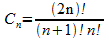# Numb3rs 503: Blowback

In this episode, the LAPD is investigating the murder of 8 people in a diner, with the help of Charlie. One of the victims was an off-duty cop, and another was undercover. The team begins to look into the daily routines of the cops on duty: where they were, what calls they answered. They bring up the idea of a 3-D stereogram, a picture within a picture. Applying this model, they see that while it appeared that all the 911 calls answered by the cop were random, in fact there was a call from the same house every week at the same time, and even though it wasn’t in the cop’s district, he was always the closest at the time of the call and thus always answered it.

What the FBI agents were looking for was a pattern within a pattern, looking at a seemingly random distribution of calls that were answered by the cop over the course of months, and finding that a subset of them in fact were quite predictable. Mathematicians often look for patterns hidden in things that appear random. We want to look at one example of this.

Pick a whole number, call it n. Then we can consider a list of the numbers 1, 2, … , n. If we write the numbers in a different order, we call this a permutation. We can think of this as picking a number from the list and writing it down, then picking a second from those that are left, then a third, and so on. For easier reading, we write 12 to mean “the permutation 1,2,” not twelve. This is fine since all the numbers we will use are less than ten.

 EXAMPLE The permutations of n=2 are 12 and 12.

• Make a list of all the permutations of 3. How many are there?

Given a permutation, we can pick out some of the terms, called a subsequence, and describe their behavior. Let’s look at a random permutation and see if we can find a specific pattern embedded into it. Say we had the permutation 7 2 5 4 3 1 6 8.

• Let’s pick out just the even terms: 2 4 6 8. What do you notice?

• Let’s now pick out just the odd terms. What subsequence do you get? Do you see a pattern?

Just by looking at the permutation, you might not have noticed that there was a structure hidden inside it. Now, we want to look at subsequences and describe their behavior. We usually do not get subsequences that are just increasing or decreasing, so we say a subsequence has a pattern where we label the lowest value in the pattern by 1, the second lowest by 2, and so on.

 EXAMPLE In the permutation 654123, let us pick out subsequence 654. Then this is a 321 pattern. Note that the numbers do not have to be sequential: the subsequence 642 also is a 321 pattern. The subsequence 613 is a 312 pattern.

• Can you find more 312 patterns in 654123?

• Can you find a 1234 pattern in 654123?

We say that 654123 contains the pattern 312, but avoids the pattern 1234.

• Make a list of all the permutations of 3 that contain the pattern 12.

• What permutations are left? These are the permutations that avoid 12.

Now let’s consider the pattern 123.

• Look at your list of all the permutations of 3. How many of these are 123-avoiding? How many are 321-avoiding?

• Here is a list of all the permutations of 4. How many of these are 123-avoiding?

 1234 2134 3124 4123 1243 2143 3142 4132 1324 2314 3214 4213 1342 2341 3241 4231 1423 2413 3412 4312 1432 2431 3421 4321

• How many are 321-avoiding?

• Can you guess how many 321-avoiding permutations of 5 there are?

It turns out that there are 42 of them! Mathematicians have studied much bigger permutations, and what is amazing is that there is a formula that tells you how many 321-avoiding permutations of n there are, for any n. In fact, this is the same number of 123- avoiding, and the same number as any π-avoiding permutations of n, for π equal to any permutation of 3. This is called the nth Catalan number, and for any n it is given by the formulawhere n!=(n)(n-1)(n-2)…(2)(1) is called n factorial.

• Check that for n=3 and n=4, this formula matches the numbers you got before.

 EXAMPLE For n= 3, 4, 5,…, we get that Cn= 5, 14, 42, 132, 429, 1430, 4862, 16796, 58786, 208012, 742900, 2674440, 9694845, 35357670, 129644790, 477638700, 1767263190, 6564120420, 24466267020, … Calculating these from a formula is much easier than working them all out by hand!